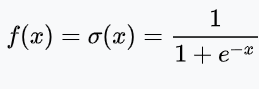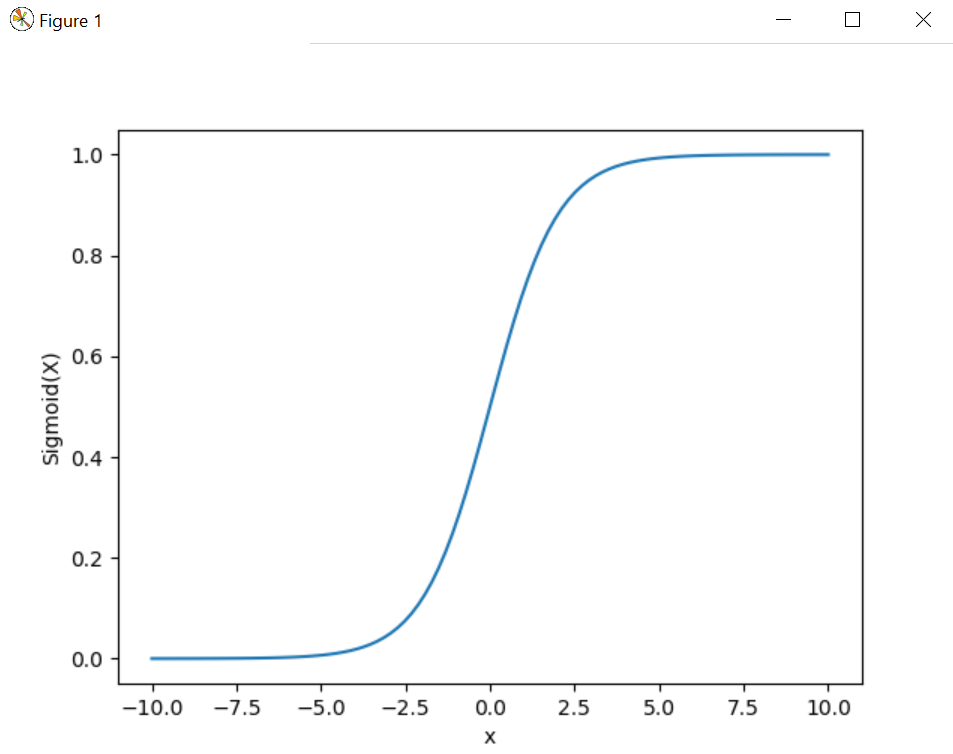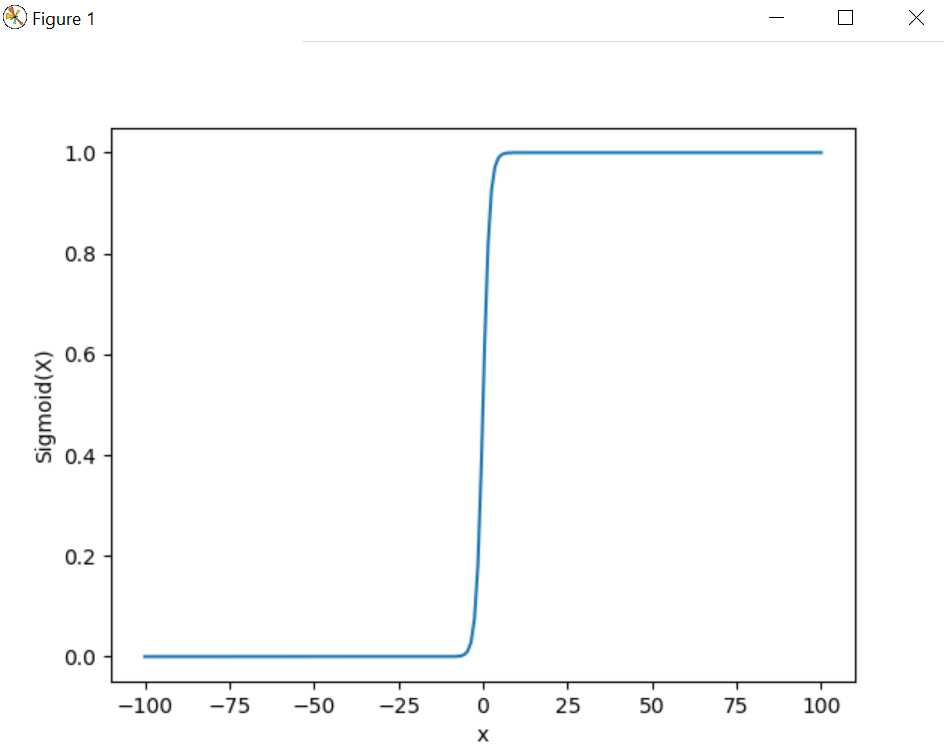# Implement sigmoid function using Numpy

With the help of Sigmoid activation function, we are able to reduce the loss during the time of training because it eliminates the gradient problem in machine learning model while training.`# Import matplotlib, numpy and math ` `import` `matplotlib.pyplot as plt ` `import` `numpy as np ` `import` `math ` ` `  `x ``=` `np.linspace(``-``10``, ``10``, ``100``) ` `z ``=` `1``/``(``1` `+` `np.exp(``-``x)) ` ` `  `plt.plot(x, z) ` `plt.xlabel(``"x"``) ` `plt.ylabel(``"Sigmoid(X)"``) ` ` `  `plt.show() `

Output :Example #1 :

 `# Import matplotlib, numpy and math ` `import` `matplotlib.pyplot as plt ` `import` `numpy as np ` `import` `math ` ` `  `x ``=` `np.linspace(``-``100``, ``100``, ``200``) ` `z ``=` `1``/``(``1` `+` `np.exp(``-``x)) ` ` `  `plt.plot(x, z) ` `plt.xlabel(``"x"``) ` `plt.ylabel(``"Sigmoid(X)"``) ` ` `  `plt.show() `

Output :My Personal Notes arrow_drop_upCheck out this Author's contributed articles.

If you like GeeksforGeeks and would like to contribute, you can also write an article using contribute.geeksforgeeks.org or mail your article to contribute@geeksforgeeks.org. See your article appearing on the GeeksforGeeks main page and help other Geeks.

Please Improve this article if you find anything incorrect by clicking on the "Improve Article" button below.

Article Tags :

Be the First to upvote.

Please write to us at contribute@geeksforgeeks.org to report any issue with the above content.